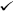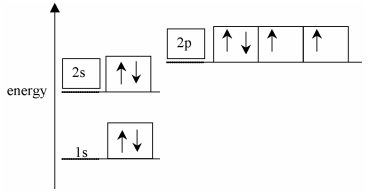1)    Define the term first ionisation energy.  

the energy required to remove one electronfrom each atom in one moleof gaseous atoms2)    Electrons are arranged in energy levels.

Complete the table below to show how many electrons completely fill each of the following.  

 number of electrons a d orbital 2 a p sub-shell 6 the third shell (n = 3) 18

3)    The energy diagram below is for the eight electrons in an oxygen atom. The diagram is incomplete as it only shows the two electrons in the 1s level.

Complete the diagram for the oxygen atom by:

(a)      adding labels for the other sub-shell levels,  

(b)     adding arrows to show how the other electrons are arranged.4)    The table shows the first 6 successive ionisation energies of an element X, which is in Period 3 of the Periodic Table.

Use the table to identify element X. Explain how you decided on your answer.  

 element ionisation energy / kJ mol–1 1st 2nd 3rd 4th 5th 6th X 578 1817 2745 11 578 14 831 18 378

AlSharp rise in successive ionisation energy between 3rd and 4th IEmarking a change to a new or different shell / there are 3 electrons in the outer shell5)    (a)      Write an equation, with state symbols, to represent the second ionisation energy of calcium.  

Ca+(g) ® Ca2+(g) + e−            Equation with correct charges and 1 electron loststate symbols(b)      Why is the second ionisation energy of calcium greater than their first ionisation energies?  

same number of protons or same nuclear charge attracting less electrons / electron removed from an ion / ion is smaller(c)     Explain why the first ionisation energy of strontium are less than that of calcium.  

atomic radii of Sr > atomic radii of Ca / Sr has electrons in shell further from nucleus than Ca / Sr has electrons in a higher energy level / Sr has more shellsTherefore less attractionSr has more shielding than Ca(‘more’ is essential)

increased nuclear charge is outweighed / despite increased nuclear
charge …..by at least one of the factors above6)    The electronic configuration of a bromine atom can be written in terms of sub-shells.

(a)      Complete the electronic configuration of a bromine atom.  

1s22s22p63s23p6 ...............

1s22s22p63s23p6..........3d104s24p5Award 1 mark for p5.

(b)      Why is bromine classified as a p-block element?  

Highest energy sub-shell/sub-shell/being filled is the p sub-shell/outer electrons are in a p (sub-shell/orbital/shell)(c)    Write the electron configuration for a bromine ion  

1s22s22p63s23p6 ...............

1s22s22p63s23p6..........3d104s24p6